• No products in the cart.

# 204.7.7 Boosting

##### Concepts behind Boosting.

Link to the previous post : https://statinfer.com/204-7-6-practice-random-forest/

In this post, we will cover how boosting work and the type of boosting algorithms.

## Boosting

• Boosting is one more famous ensemble method.
• Boosting uses a slightly different techniques to that of bagging.
• Boosting is a well proven theory that works really well on many of the machine learning problems like speech recognition.
• If bagging is wisdom of crowds then boosting is wisdom of crowds where each individual is given some weight based on their expertise.
• Boosting in general decreases the bias error and builds strong predictive models.
• Boosting is an iterative technique. We adjust the weight of the observation based on the previous classification.
• If an observation was classified incorrectly, it tries to increase the weight of this observation and vice versa.

### Boosting Main idea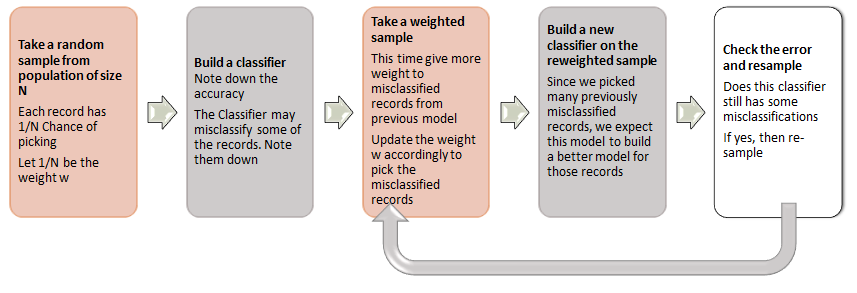Final Classifier C=αici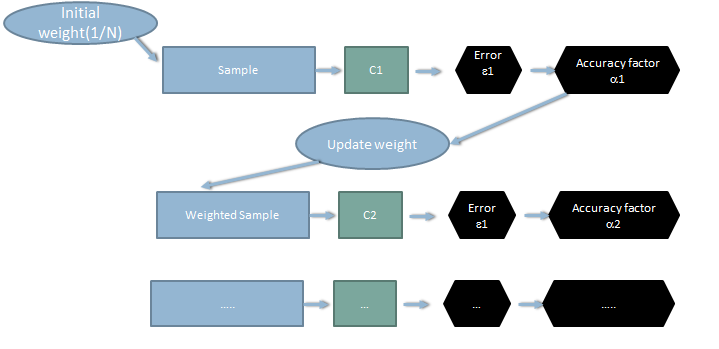### How weighted samples are taken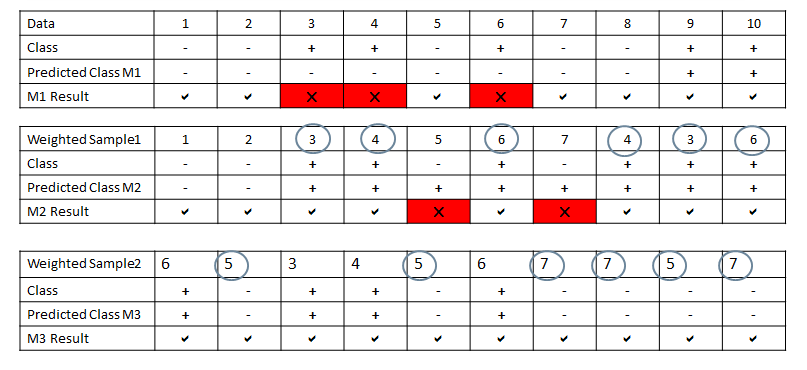### Boosting Illustration

Below is the training data and their classes We need to take a note of record numbers, they will help us in weighted sampling later.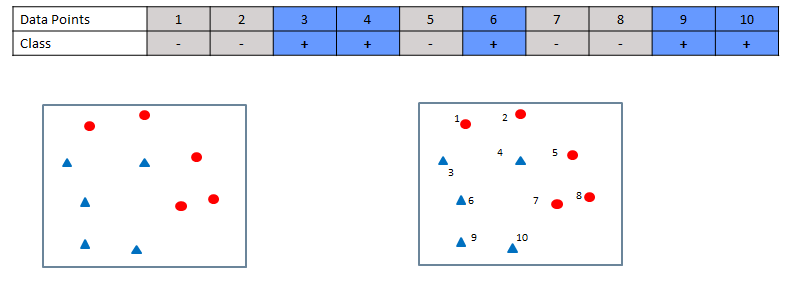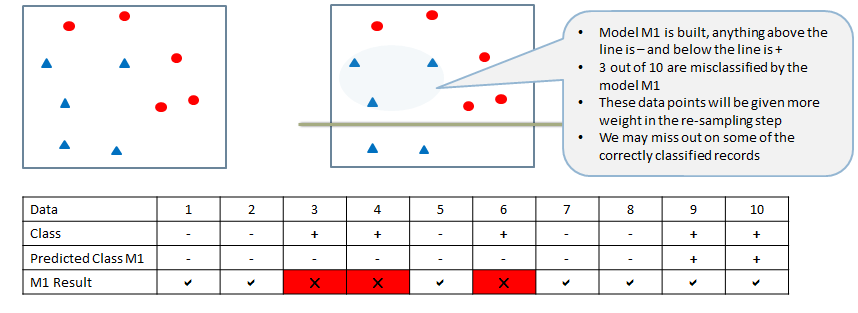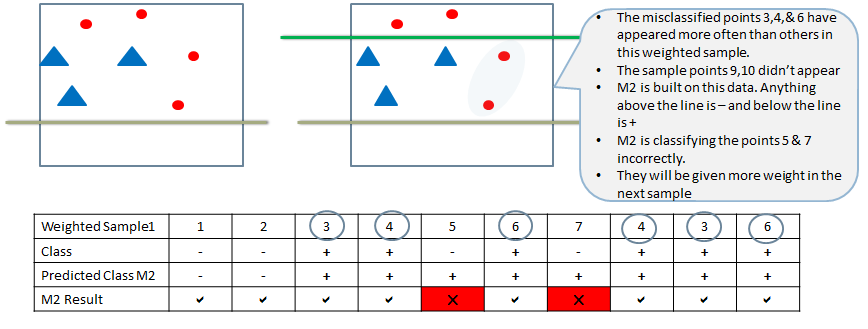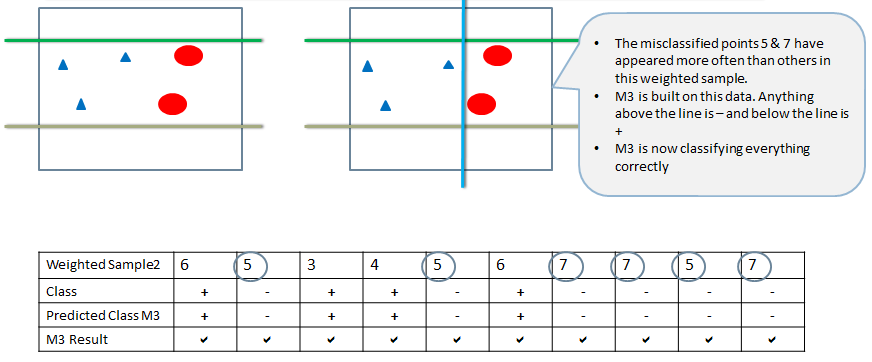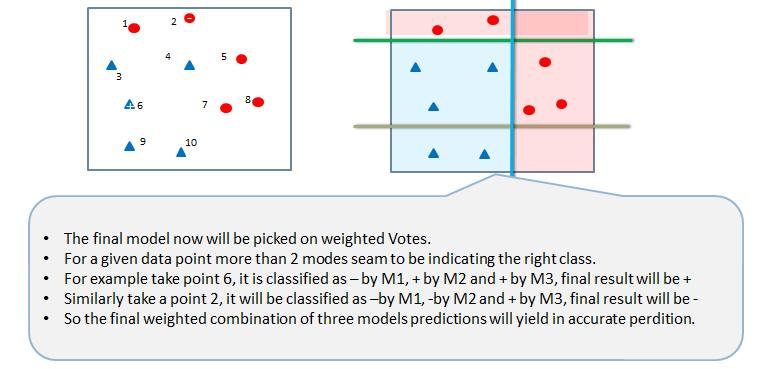### Theory behind Boosting Algorithm

• Take the dataset.
• Build a classifier Cm and find the error.
• Calculate error rate of the classifier
• Error rate of= Sum of misclassification weight / sum of sample weights
• Calculate an intermediate factor called a. It analogous to accuracy rate of the model. It will be later used in weight updating. It is derived from error.
•• Update weights of each record in the sample using the a factor. The indicator function will make sure that the misclassifications are given more weight.
• For i =1,2,… N
•• Re-normalize so that sum of weights is 1.
• Repeat this model building and weight update process until we have no misclassification.
• Final collation is done by voting from all the modes. While taking the votes, each model is weighted by the accuracy factor α
•• Adaptive Boosting -Till now we discussed Ada boosting technique. Here we give high weight to misclassified records.